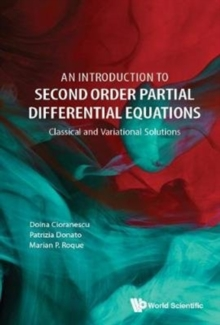Supporting your high street Find out how »
• My Account# Introduction To Second Order Partial Differential Equations, An: Classical And Variational Solutions Hardback

#### Description

The book extensively introduces classical and variational partial differential equations (PDEs) to graduate and post-graduate students in Mathematics.

The topics, even the most delicate, are presented in a detailed way.

The book consists of two parts which focus on second order linear PDEs.

Part I gives an overview of classical PDEs, that is, equations which admit strong solutions, verifying the equations pointwise.

Classical solutions of the Laplace, heat, and wave equations are provided.

Part II deals with variational PDEs, where weak (variational) solutions are considered.

They are defined by variational formulations of the equations, based on Sobolev spaces.

A comprehensive and detailed presentation of these spaces is given.

Examples of variational elliptic, parabolic, and hyperbolic problems with different boundary conditions are discussed.

#### Information

• Format: Hardback
• Pages: 300 pages
• Publisher: World Scientific Publishing Co Pte Ltd
• Publication Date:
• Category: Differential calculus & equations
• ISBN: 9789813229174

£69.00

£57.15